# 图像分割(Segmentation)——K-Means, 最小割, 归一化图割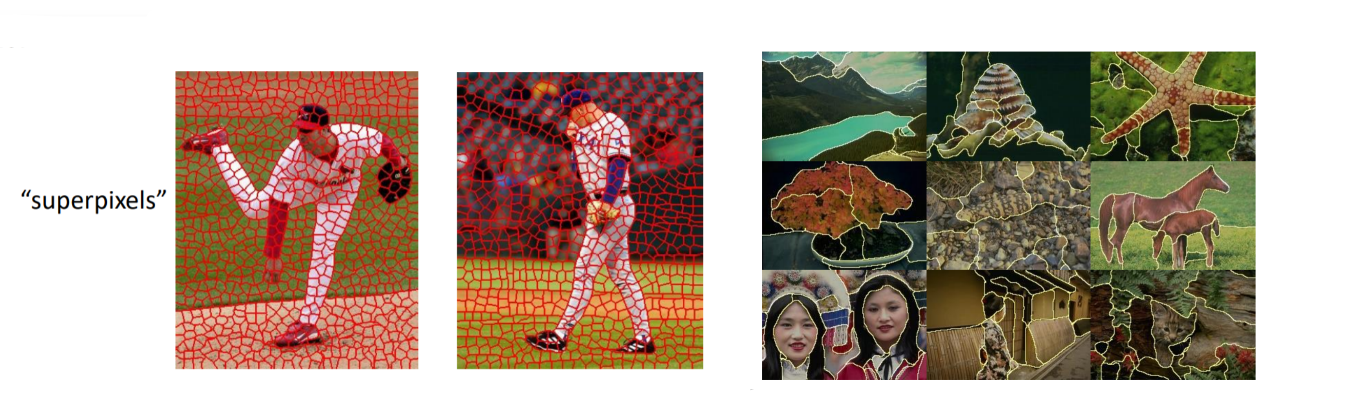## Gestalt理论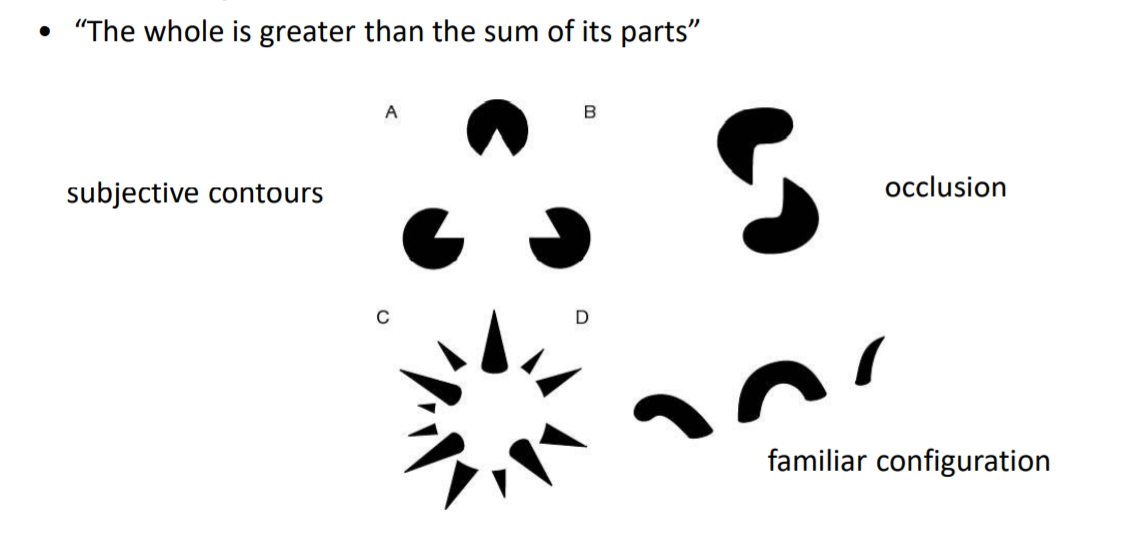Gestalt中常见的一些分组的情况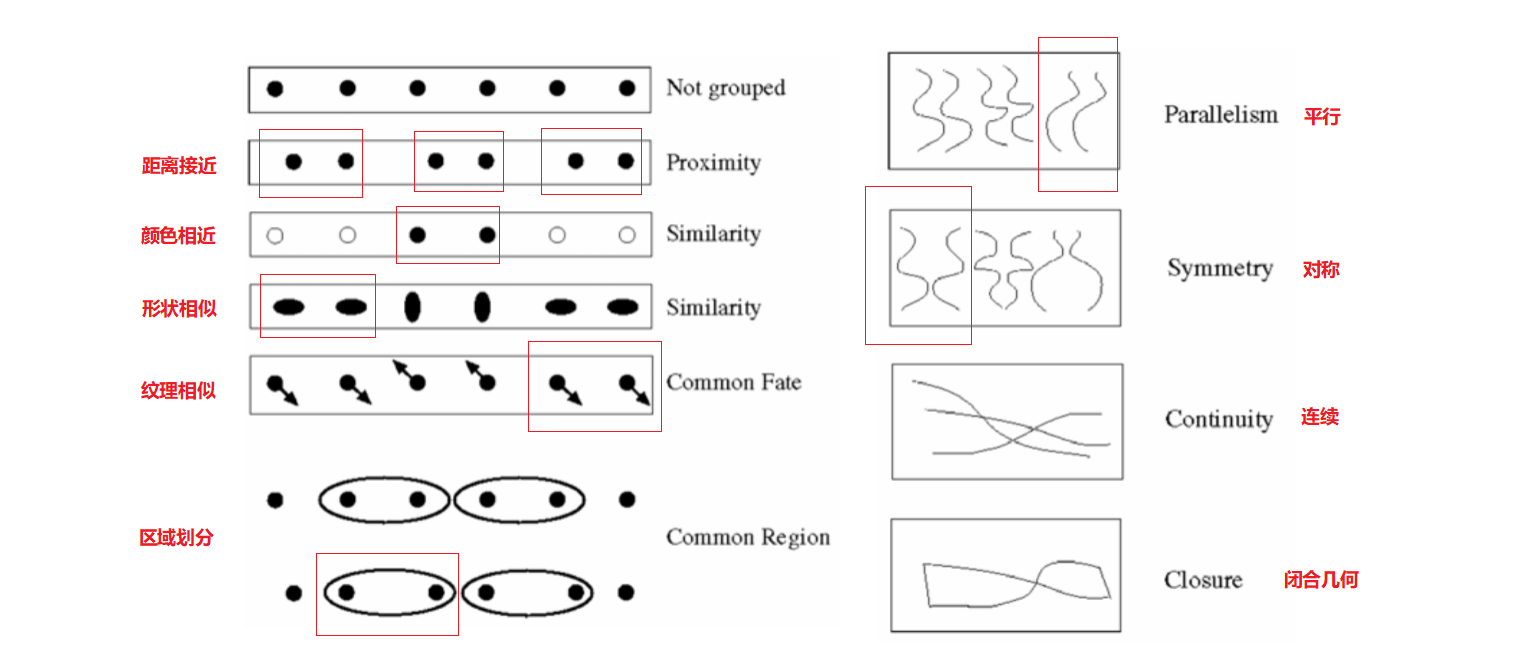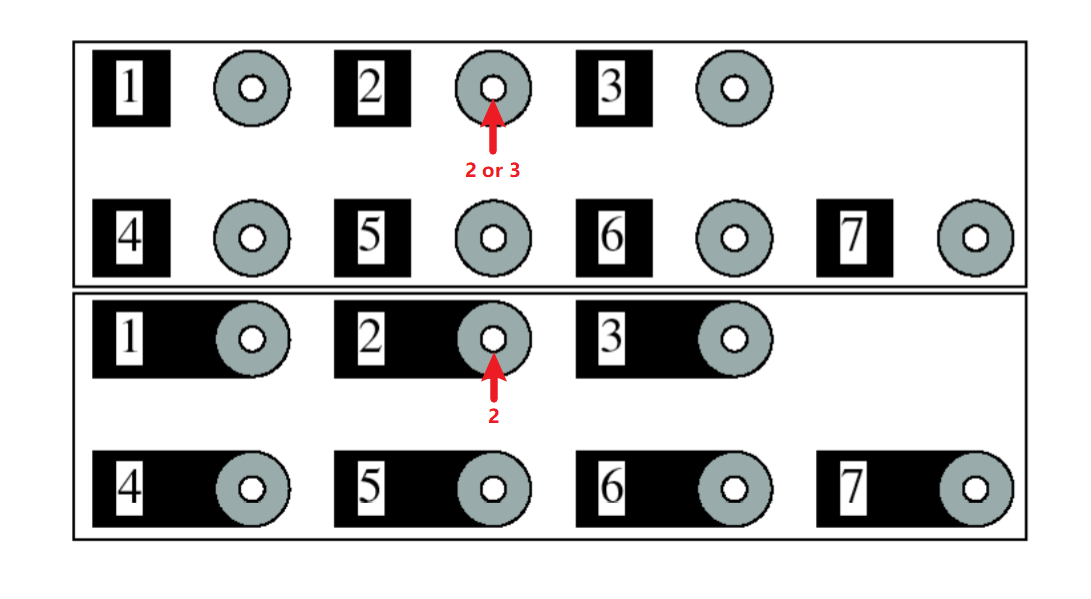## K-Means聚类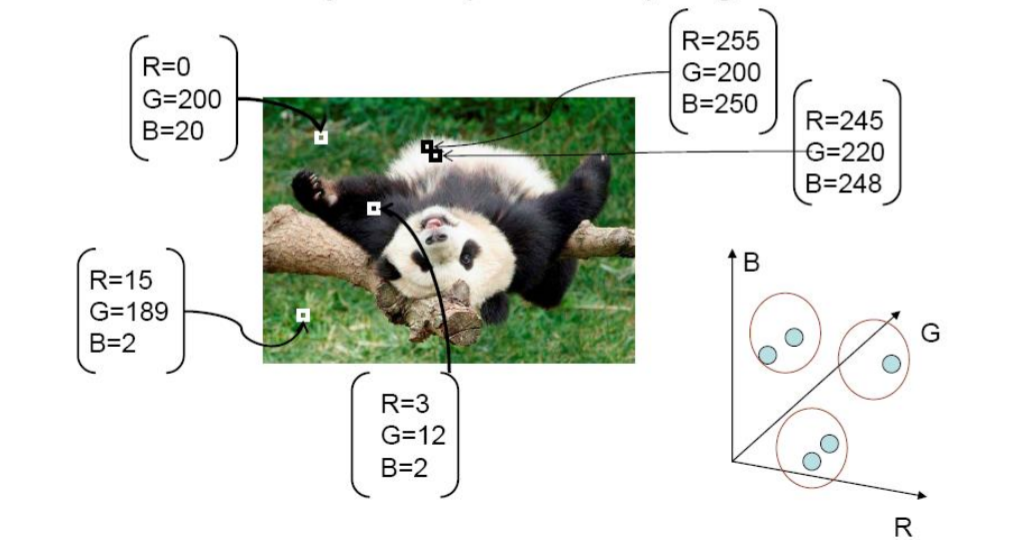K-Means算法
“物以类聚、人以群分”：

1. 首先输入k的值，即我们希望将数据集经过聚类得到k个分组。

2. 从数据集中随机选择k个数据点作为初始大哥（质心，Centroid）

3. 对集合中每一个小弟，计算与每一个大哥的距离（距离的含义后面会讲），离哪个大哥距离近，就跟定哪个大哥。

4. 这时每一个大哥手下都聚集了一票小弟，这时候召开人民代表大会，每一群选出新的大哥（其实是通>过算法选出新的质心）。

5. 如果新大哥和老大哥之间的距离小于某一个设置的阈值（表示重新计算的质心的位置变化不大，趋于>稳定，或者说收敛），可以认为我们进行的聚类已经达到期望的结果，算法终止。

6. 如果新大哥和老大哥距离变化很大，需要迭代3~5步骤。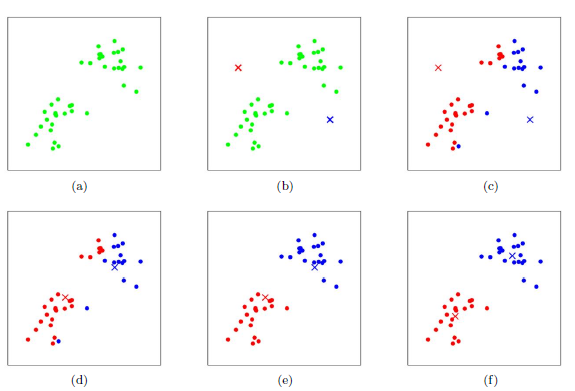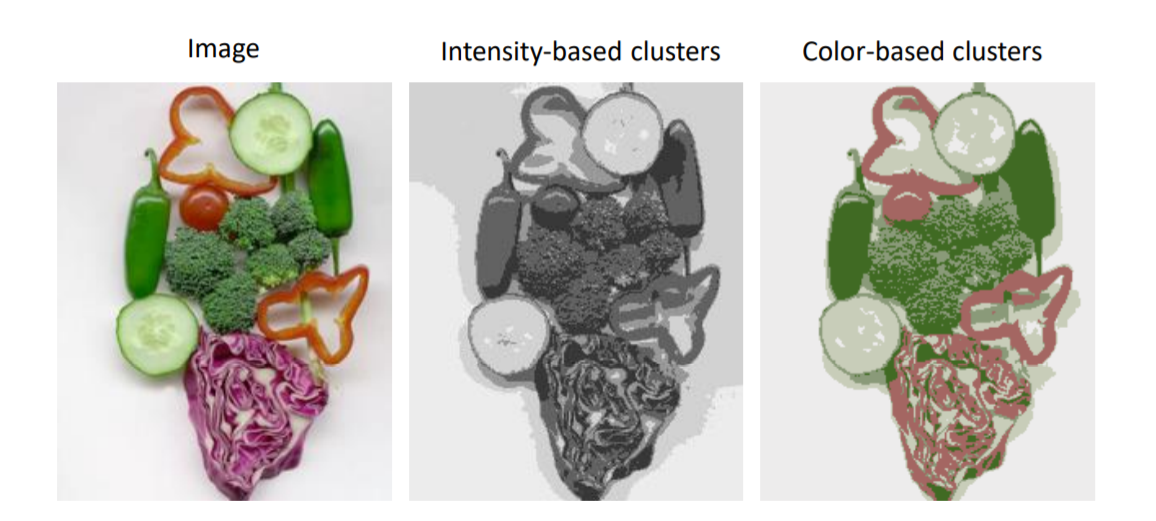• 语义分割：将不同位置上同样的物品分成同一类（如上图三）使用(R, G, B)的三维向量描述像素
• 实列分割：将不同位置上同样的物品分成不同类，此时就需要考虑像素位置对分割的影响，使用(R, G, B, x, y)的五维向量描述像素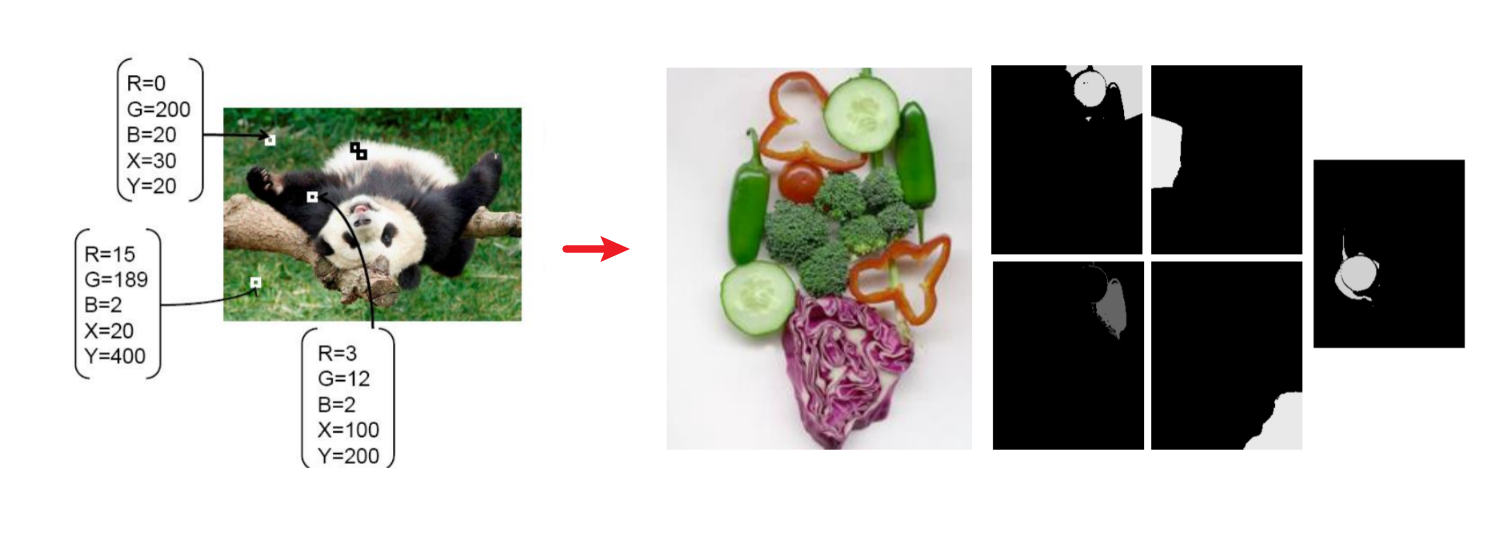## Mean shift 聚类

K-Means算法可以很简单的进行分割，但是初始值对结果的影响非常大，Mean Shift 算法处理时将像素密度的峰值作为初始值，在特征空间中寻找密度的模态或局部最大值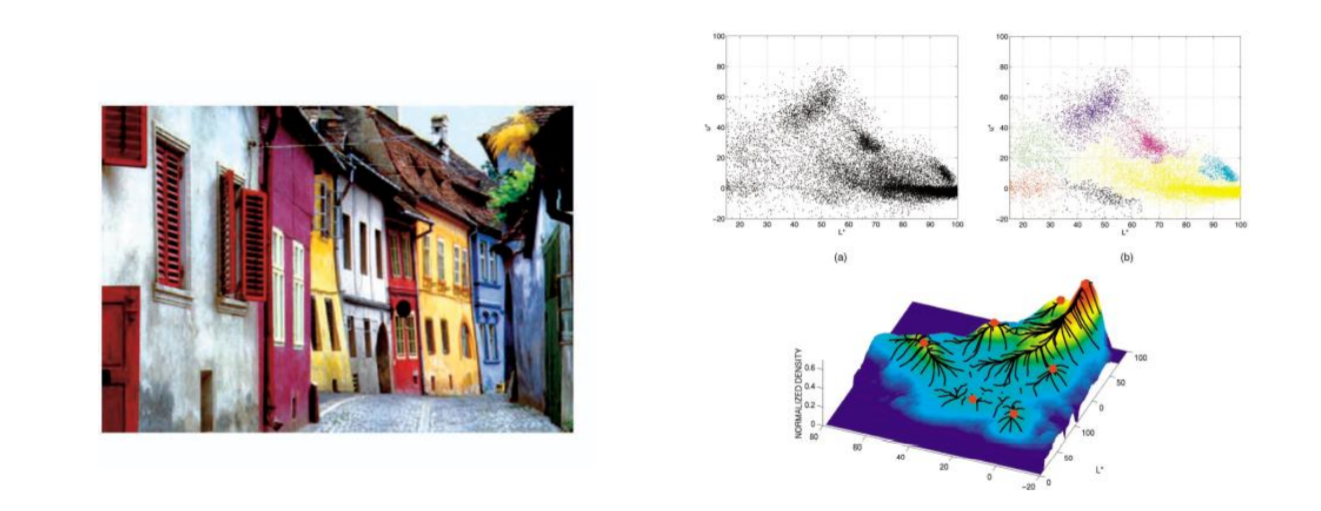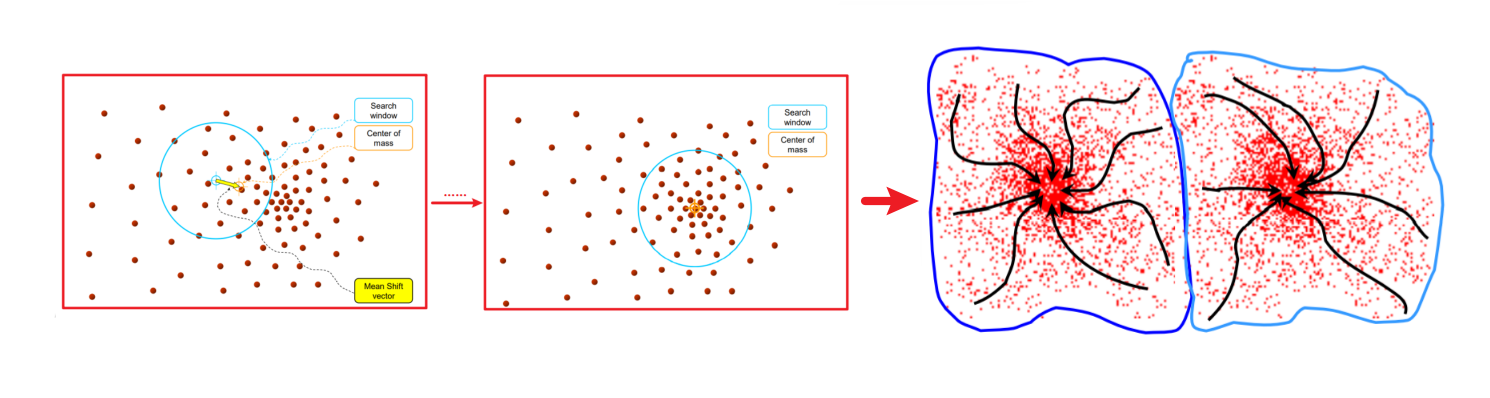2. 在单个特征点上初始化窗口
3. 对每个窗口执行Mean Shift直到收敛
4. 合并接近相同“峰值”或模式的窗口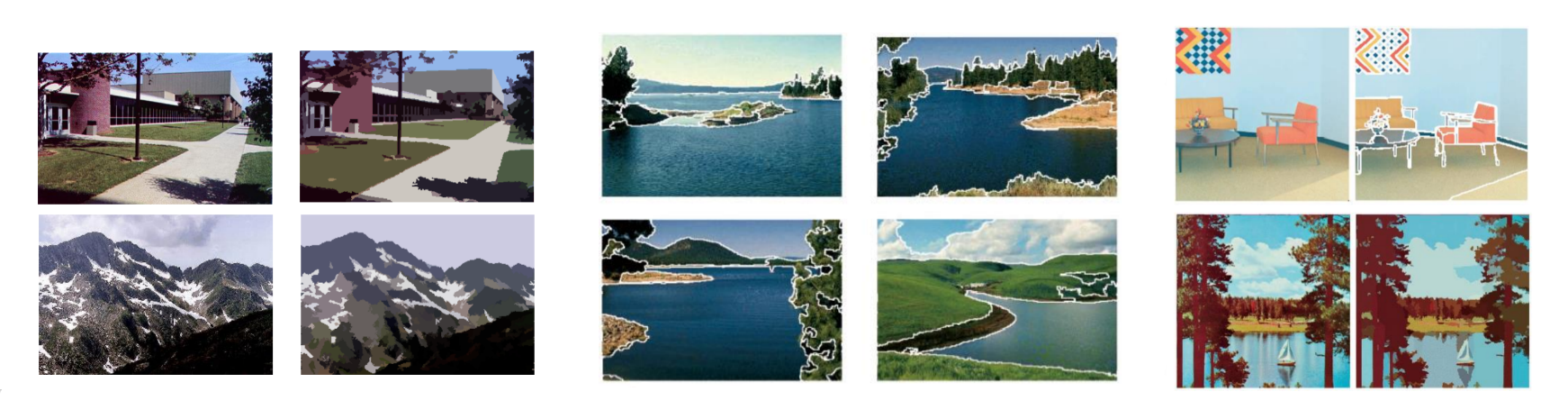• 算法计算量不大，在目标区域已知的情况下完全可以做到实时跟踪；
• 采用核函数直方图模型，对边缘遮挡、目标旋转、变形和背景运动不敏感。

• 缺乏必要的模板更新；
• 跟踪过程中由于窗口宽度大小保持不变，当目标尺度有所变化时，跟踪就会失败；
• 当目标速度较快时，跟踪效果不好；
• 直方图特征在目标颜色特征描述方面略显匮乏，缺少空间信息。

## Image as Graphs

• 节点代表像素
• 每对节点之间代表边缘(或每对“足够接近”的像素)
• 每条边都根据两个节点的亲和力或相似性进行加权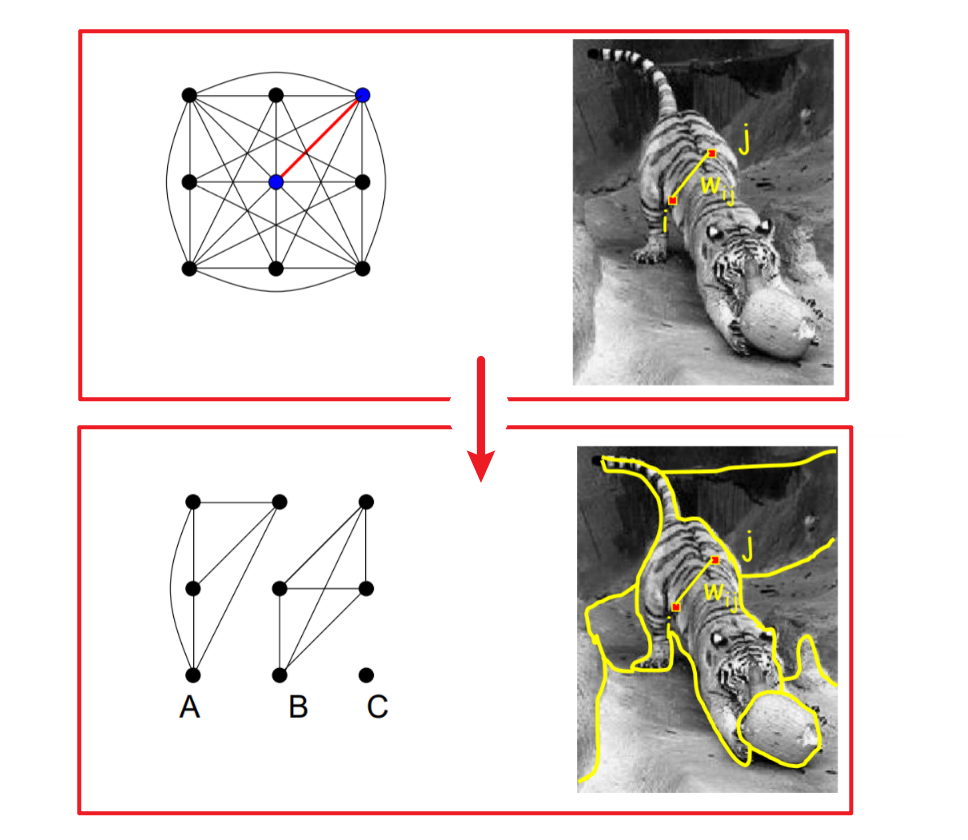• 找一条边将，将像素之间的联系切断
• 将切断的边的权重相加，使和最小（相似的像素应该在相同的部分，不同的像素应该在不同的部分）

$exp(-\frac{1}{2\sigma^2}dist(X_i,X_j)^2)$

1. 假设用特征向量x表示每个像素，并定义一个适合于该特征表示的距离函数: $dist(X_i,X_j)^2$
2. 然后利用广义高斯核将两个特征向量之间的距离转化为相似性
3. 小的$\sigma$：仅群聚邻近点；大的$\sigma$：群聚距离远的点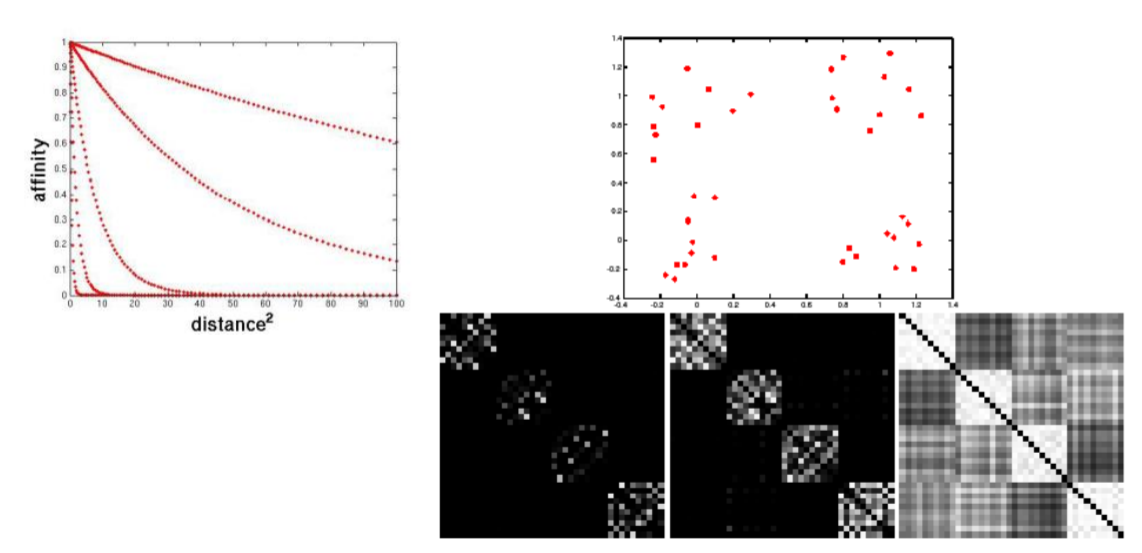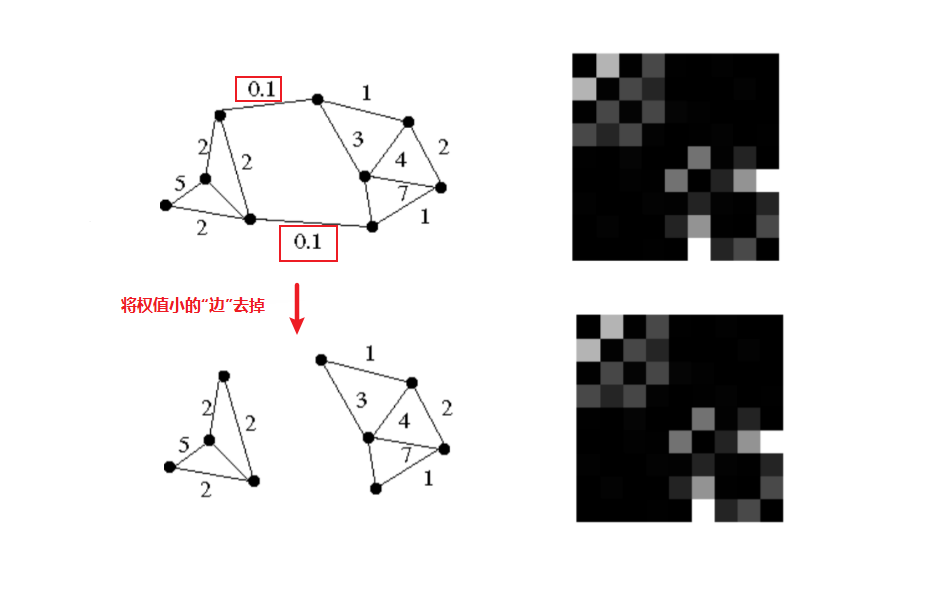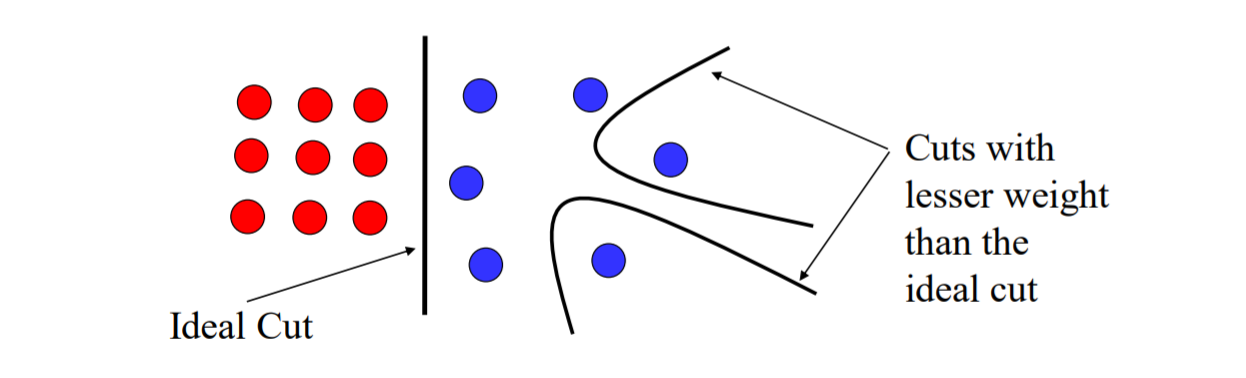$\frac{W(A,B)}{W(A,V)}+\frac{W(A,B)}{W(B,V)}$

• $W(A,B)$：表示集合$A, B$中所有边的权重的和
• $W(A,V)，W(B,V)$：分别表示分割后集合$A, B$内边的个数，当总的边数一定，其中一个$W$很大时，另一个就会很小，导致整个算式所计算的权重就会很大
• 定义一个邻接关系矩阵$W$$(i,j)$表示第i个像素与第j个像素之间的权重，是一个对称的矩阵

• 定义矩阵$D$，是一个对角的矩阵$D_{ii} = \Sigma_jW(i, j)$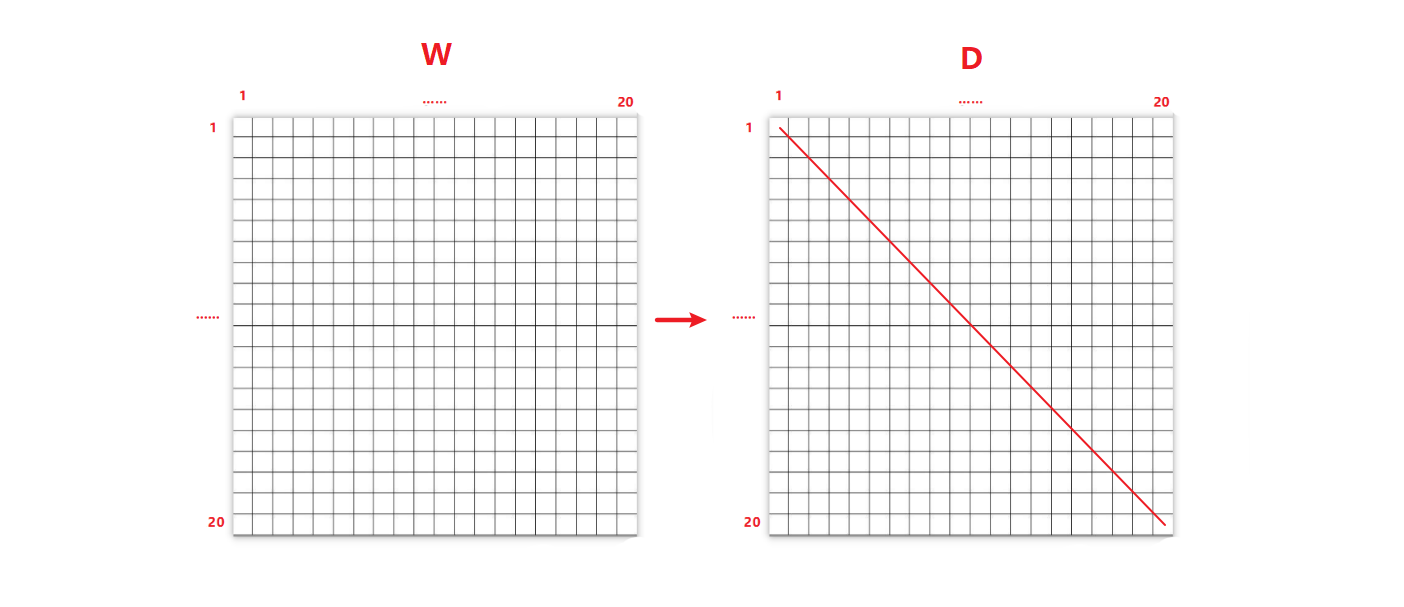$\frac{y^T(D-W)y}{y^TDy}$

• 归一化图割权重计算：其中，y是指示向量，如果第i个特征点属于A，那么它在第i个位置的值应该为1，反之则为0；利用拉格朗日算法求权重第二小（最小的是0）的特征值对应的特征向量y：$(D-W)y=\lambda y$
所求出的y不是[0,…,1,0,0…]而是存在小数的向量，需要设置一个门限，将大于门限的设置为1，小于门限的设置为0

• 使用特征向量y的项对图进行二分割

• 改变门限，迭代将图像分成多个区块，也可以使用K-Means将得到的特征向量分类，达到分割多块的效果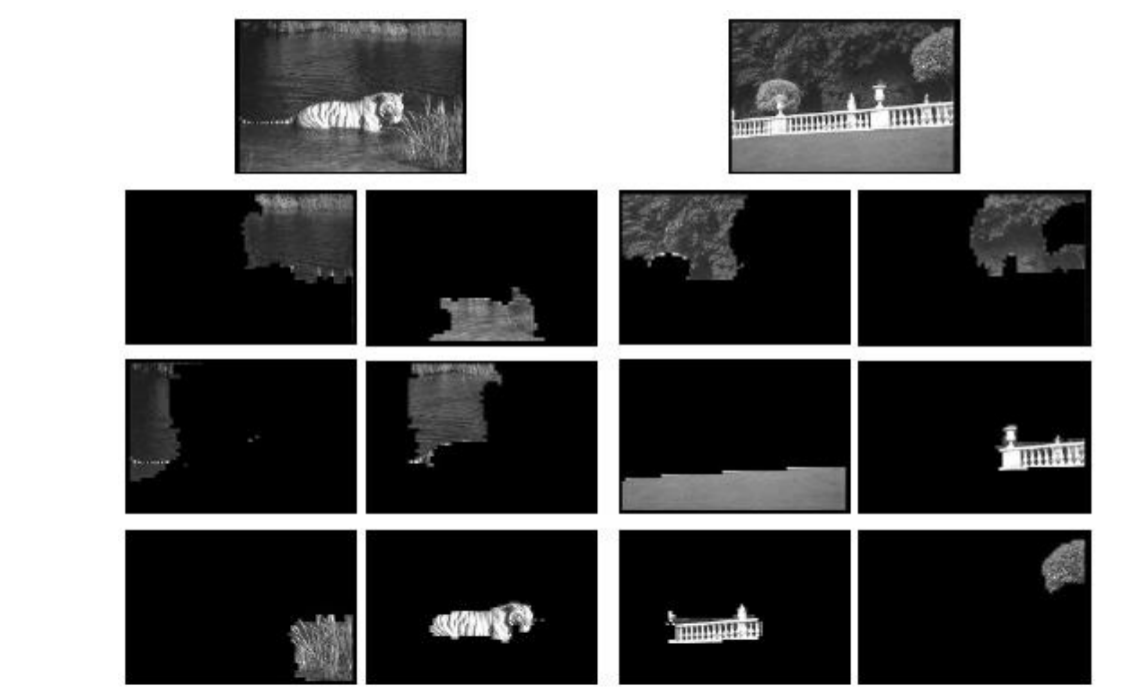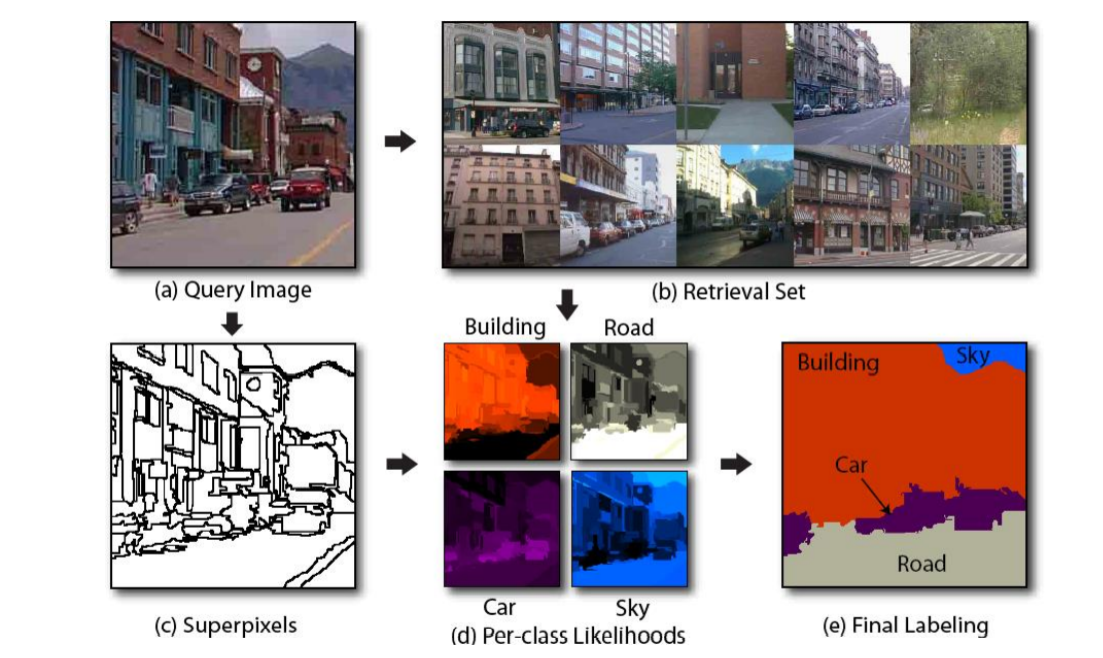posted @ 2020-07-31 18:13  Moonx5  阅读(1109)  评论(0编辑  收藏  举报